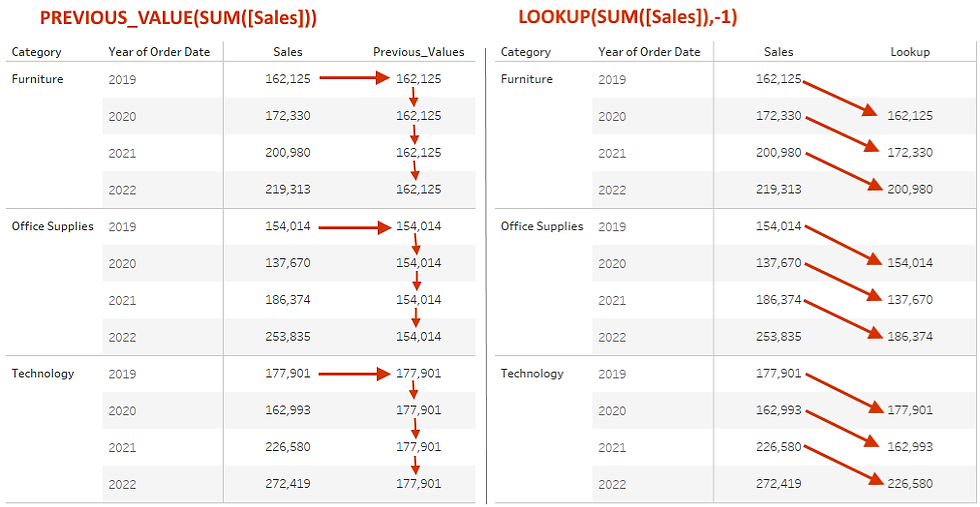top of page
Search
• Bernard K

# PREVIOUS_VALUE () Function in Tableau### Introduction

PREVIOUS_VALUE function returns the value of this calculation in the previous row. Returns the given expression if the current row is the first row of the partition.

In this article, I will be exploring how this function differs from LOOKUP function, as well as other ways you can apply this function.

• PREVIOUS_VALUE vs LOOKUP function

• Computing running sum

• Computing running product

### PREVIOUS_VALUE Vs LOOKUP function

While you might think PREVIOUS_VALUE is the right function to use - to return the previous values of your field – you might be surprised that it can’t. (The right function to return the previous values in your field is the LOOKUP function with an offset of -1). The previous value is computed a little-bit different.

The difference between these two functions can be illustrated as shown in the view below.

(When computed table-down)The same functions return the following when computed pane-down.As illustrated above, the previous_value function can be much helpful when replacing gaps in your data with the previous value in the partition. For example, the gaps in the first image below - can be replaced with the previous value using the following calculation.Adding the above calculation to the view - replaces the gaps with the previous value as shown below.### Computing running sum

The previous_value function can also be used to replicate the running sum calculation using the calculation below.

Running SUM = SUM([Sales]) + PREVIOUS_VALUE(0)

This calculation takes the sum of sales from the current row and adds that to the value of this calculation in the row above. For the first row, the sum of sales is added to zero – which returns the sales for January = 96,070.### Computing running product

The previous_value function can also be used to compute a running product calculation using the formula below.

Running Product = SUM([Discount]) * PREVIOUS_VALUE (1)

This calculation takes the Discount value of the current row and multiplies that with the results of this calculation in the row above. For the first row, the Discount value of the current row is multiplied by 1, resulting to Discount for January = 57.1(This calculation is executed same as the running total, only this time using multiplication as the operator)

### Conclusion